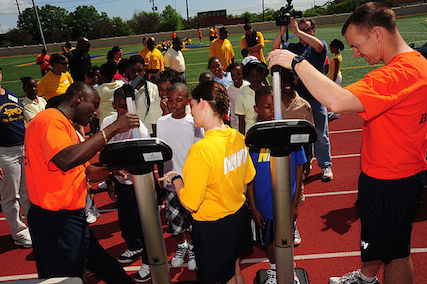# Principal Nigel's Math Question

Algebra Level 1Principal Nigel wanted to figure out the height of the 7th graders, and transform it into a problem for the 6th graders. After hours, of hard work the 6th graders couldn't figure it out. Can you help them? Well here it is:

7th graders' average height for the girls is 54 inches. The average height for the boys is 55 inches. If the ratio of girls to boys is 7 to 6, what is the average height of all the students?

×